U.S. Department of Transportation
1200 New Jersey Avenue, SE
Washington, DC 20590
202-366-4000

Federal Highway Administration Research and Technology
Coordinating, Developing, and Delivering Highway Transportation InnovationsThis report is an archived publication and may contain dated technical, contact, and link information
 Federal Highway Administration > Publications > Research Publications > Geotechnicals > 03089 > Dynamic Bridge Substructure Evaluation and Monitoring
 Publication Number: FHWA-RD-03-089 Date: September 2005

# Dynamic Bridge Substructure Evaluation and Monitoring

## Chapter 7. Using the Hilbert-Huang Transform for Substructure Damage Evaluation

### Background

Because of the failure of the structural parameter estimation approach to identify substructure damage, the completion of this research project was delayed to conduct a preliminary evaluation of the capabilities of a comparatively new technique, the HHT. The premise of this research is that identifying certain vibration patterns (or signature recognition) by data analysis of vibration records can reveal structural dynamic characteristics such as natural frequencies and modes. This approach in structural engineering already has broad-based applications such as structural model validation and updating, condition assessment and monitoring, and damage diagnosis and detection. Traditional modal analysis generally has been found lacking in identifying damage in structures until failure was imminent. This is caused by the redundancy present in most structures and the difficulty in identifying the change in response to lower frequencies and less damping of an individual member without directly exciting and monitoring the member. In other words, the shift from a linearly elastic response to a nonlinear, damaged response generally is not apparent in the modal vibration measurements and analyses until failure is just about to occur or has occurred.

The HHT technique has the potential to address this problem because it was researched and developed to use modal vibration measurement data to identify nonlinear, damaged response of members. In this research, the HHT technique was applied to bents 12 and 2 of structure 4 of the Trinity River Relief Bridge as an alternative to the signature recognition of modal vibration analyses used with Fourier-based or wavelet data processing. This chapter reports the results of a preliminary evaluation of the HHT method to identify substructure damage signature from vibration data. In particular, HHT analyses were performed on the intact, excavated, and damaged pile foundations of bents 12 and 2.

The interpretation of the HHT analysis for the vibration records is based on the following observations, assumptions, and theories of vibration and HHT:

• Structural vibration to a given excitation should reveal its proper frequency content, which includes driving frequencies, natural frequencies of the whole structure or local members, or both, and noise frequencies.
• When a member of the structure is damaged, its stiffness is reduced in comparison with its undamaged state; therefore, vibration recorded at the damaged member could show larger vibration amplitude at lower frequency than the vibration at the same member without damage.
• Even though the damage, or the stiffness reduction, to the local structural member is large with respect to the member, it still might be small in comparison with the whole structural stiffness. Consequently, vibration recorded on the other members might not be sensitive to such a localized damage. In other words, for damaged or undamaged structures, vibrations at a member other than the to-be-damaged or damaged member will show almost the same vibration amplitude at almost the same natural frequencies.
• Because the HHT method can identify the instantaneous frequency of measured vibrations through the Hilbert spectrum, the frequency of vibration for a damaged member could be observably less than the frequency on other members. This leads to identifying the damaged structural member.

The HHT analyses of the testing data on bents 12 and 2 were found to support these assertions.

As a prelude to the use of the HHT technique, the following paragraphs review the current nondestructive techniques and give an overview of the limitations of current vibration-based signature-recognition techniques. Following that is a discussion of the limitations of the modal vibration analysis approach described in chapter 5. Further discussion describes the HHT method and its application to bents 12 and 2. This chapter concludes with the proposed approach for further research and application of the HHT to dynamic evaluation of bridge substructures.

### Current Approaches in Nondestructive Condition Assessment

Regular condition assessment inspections are essential for reliable performance of aging and deteriorating civil structures and infrastructure, as described by Mori and Ellingwood(139,140) and Olson and Wright.(141) Currently, bridge engineers and inspectors must visually examine each visible major member and certify its safety, particularly after natural hazards such as an earthquake. Such a visual inspection approach is limited to surface conditions; it is neither totally objective nor reliable because of the great uncertainty in identifying the existence, location, and degree of internal damage, as described by Doebling et al.(50,142,143) This costly and ineffective approach stimulated recent research and development in new technologies for the NDE of civil infrastructures, described by Chang(144) and Chase and Washer.(145) These new technologies are in the areas of infrared thermography, ground-penetrating radar, acoustic emission monitoring, and eddy current detection. While these technologies are feasible technically, they probably are used most efficiently when the locations of damage are first identified. For a complicated structure, locating the damage is difficult. Some members are physically unreachable or invisible, or both; a large number of sensors are required; and a large instrumentation system is costly, requires frequent recalibration, and involves extensive data analysis. The foundation elements of bridge substructures are not visible at all; consequently, visual inspection is not useful unless a failure also affects the visible bridge elements.

Using only stress-wave and vibration-based measurements is sometimes a simpler, less costly technique, as described by Olson and Wright,(141) Farrar et al.,(33,146) Farrar and Doebling,(147) Salawu and Williams,(42,148) Salawu,(149) Caicedo et al.,(150) and Chang.(151) These techniques, however, can lead to large, costly instrumentation systems. Moreover, without appropriate data analysis methods, they will not identify damage precisely, and access must be gained to each of the critical members, also costly and time consuming.

### Current Vibration-Based Signature-Recognition Techniques

Identification of certain vibration patterns (or signature recognition) from vibration records can reveal structural dynamic characteristics such as natural frequencies and modes. This ability to identify vibration patterns is of paramount importance to broad-based applications in structural engineering such as structural model validation and updating, condition assessment and monitoring, and damage diagnosis and detection. With a few exceptions, two sets of large-array vibration data recorded at multiple points (pre- and post-damage) are usually required. Analysis and comparison of these two data sets can reveal different dynamic characteristics in the structure, and thus lead to the identification of damage location and severity. This kind of approach builds on the incorporation of the experimental data process with simplified structural modal models, described by Caicedo et al.,(150) Chang,(151) Aktan et al.,(152) Hou et al.,(153) Kim and Stubbs,(154) Park et al.,(155) Shi et al.,(156,157) Shah et al.,(158) Smyth et al.,(159) Vanik et al.,(160) Sohn et al.,(161) Vestroni and Capecchi,(162) and Yun et al.(163) The currently used signaturerecognition methods can be improved upon in three respects, which are covered in the following subsections.

### Experimental Vibration Data Processing Analysis Approaches

Traditional data processing such as Fourier-based provides distorted or indirect information of nonstationary data of measured vibrations (such as from traffic) that are also nonlinear for a damaged structure. This can mask real effects of the damage and mislead those using the data for signature recognition.

For example, the Fourier spectral analysis approach used in this study is one of the most widely used methods for vibration data analysis. It is valid, however, only for processing linear, stationary data. The Fourier spectrum defines harmonic components globally. That is, it yields average characteristics over the entire time duration of the data; however, some characteristics of vibration data such as the dominant frequencies may change significantly over different portions of the duration. Also, because Fourier spectral analysis explains data in terms of a linear superposition of harmonic functions, it typically needs higher frequency harmonics to simulate nonlinear, nonstationary data. In short, Fourier spectral analysis distorts information from data by spreading the energy over a wide frequency range and losing the locality of energy distribution, that is, the location of the damaged members.

To improve the Fourier approach, windowed Fourier spectral analysis can be used. In this approach, Fourier spectral analysis is performed over restricted windows centered on specific features of interest in the data. The analysis provides information on frequency content locally in the data, thus reducing the problem of nonstationary data, although still not solving for the nonlinear issue. The window technique reduces frequency resolution as the length of the window shortens, thus requiring a tradeoff: the shorter the window, the better the locality of the Fourier spectrum, but the poorer the frequency resolution. In addition, selection of window length is primarily visual and subjective.

Wavelet analysis has been used in past decades to recover more accurate local information from nonstationary data. It has found applications in, for example, earthquake data processing,(164, 165,166) system identification,(153,167) and random field representation.(168,169) According to Daubechies, among many others, wavelet analysis can provide a sound tool in situations where better time resolution at high frequencies rather than at low frequencies is desirable.(170) On one hand, the wavelet analysis can characterize time-dependent high-frequency signals effectively from a vibration record. This feature is particularly useful to single out, from a time-vibration record, abnormal high-frequency signals that are likely related to one type of structural damage, such as cracks during a severe earthquake.(153)

On the other hand, wavelet analysis does not result in any essential improvements over conventional or windowed Fourier spectral analysis to characterize low-frequency signals from a record, because the largest scale in the wavelet transform (related to the frequency resolution) should be less than one-half of the length of the entire time series.(171) The difference in lowfrequency signals of the vibration records in a damaged structure from those in the undamaged structure may indicate another type of structural damage such as continuous degradation of a structure from corrosion and other deterioration mechanisms. Wavelet transform is strongly conditional on the selected mother wavelet; consequently, the physical interpretation of each wavelet decomposition and spectrum is indirectly related to the underlying physical process that the data exposes through the different mother wavelets, explained in an example later.

### Signature of Damaged Structural Members

Modal analysis often is used with data processing of in situ vibration responses for structural identification or signature recognition. Such an approach is neither efficient nor sensitive when identifying the change of local dynamic properties of a structure from vibration records.

The modal analysis builds on multiple DOF modeling of the whole structure as discussed in chapter 6. The signature of local dynamic properties can be identified only through higher modes. Determining the shape of higher modes requires a large number of sensors, which makes data collection not only costly, but also complicated (for example, in issues on optimal locations of sensors). Typically, the number of response measurement locations is significantly less than the number of DOFs in a structural model, although this is not as much a problem in simpler substructures. This mismatch often makes it difficult to precisely identify the local dynamic properties. Even with the detailed data from large sensory systems, sensitivity is still low for identifying local dynamic properties from vibration records. The reasons are the ubiquitous noise problem and the intrinsic drawback of traditional data-analysis methods for nonlinear, nonstationary data processing.

From the perspective of damage detection, most existing signature-recognition techniques require a priori data. In other words, vibrations need to be measured from the same structure in its undamaged state. Both a priori (healthy) and damaged structure data are analyzed by using one of the traditional data processes. The two sets of data are then compared to determine the change in natural frequencies, amplitudes, and vibration modes to locate the damage and estimate its severity. Obtaining a priori data, however, is not economically practical for most existing structures.

Even if a priori data could be obtained for important structures such as large bridges, hospitals, and communication centers, the change in natural frequencies, amplitudes, and modes in a structure&rsquo;s different health or damage conditions often is inconsequential, making the identification ineffective. Moreover, because the modal models are inherently linear and time invariant, the structure to be identified must conform to those assumptions as well. Consequently, the modal-based techniques cannot efficiently reveal the nonlinear structural features caused by damage.

### In Situ Vibration Data

For real structures, the practical amount of high-strain modal vibration testing with controlled excitation and associated damage is small. In situ vibration typically is limited to a low-strain response regime because of difficulties with controlled exciting at higher strain levels. It is difficult, if not impossible, to validate the accuracy, sensitivity, and effectiveness of signaturerecognition techniques that are developed primarily on the basis of laboratory experiments and computer models.

For example, ambient vibration data often are used in signature recognition. Because ambient excitation is not measured, however, it is unknown if this excitation source provides input at the frequencies of interest or if the input is uniform over a particular frequency range. Similarly, seismic data often are used to identify the dynamic properties of damaged structures to locate the damage and estimate the severity. Because the degree of seismic-induced structural damage is primarily visual, and thus difficult to quantify precisely, pertinent assumptions must be made in the approach. These and other factors can increase significantly the uncertainties for the use of traditional signature-recognition techniques for broad-based applications in general and identified dynamic properties in particular. The problem is a lack of high-strain, even destructive, tests of a real structure with controlled excitation and damage. These destructive tests cannot be replaced with laboratory experiments and computer models because there are always inherent differences between the models and the as-built structure. These differences arise from simplifications of the boundary and support conditions, connectivity between various structural elements, unknown material properties and constitutive relationships (particularly those associated with soil and concrete), and energy-dissipation (damping) mechanisms.

### Limitations of Modal Vibration Approach for Bent 12

This discussion focuses on the limitations of traditional modal vibration analysis approaches for signature recognition in vibration records for bent 12 of the Trinity River Relief Bridge, which was the simplest bridge substructure tested. Both Fourier spectra and wavelet analysis techniques are applied.

### Review of Field Test Conditions for Structure 4 of the Trinity River Relief Bridge

This bridge had a 6.1-m (20-ft)-wide roadway consisting of 151 concrete panels directly supported by 66 concrete pile bents with a span of 5.49 m (18 ft). The nominal thickness of the concrete slab panels between the bents was 40.64 cm (16 inches). These concrete slabs were reinforced with number 7 steel bars at a spacing of 14.6 cm (5.75 inches) longitudinally and number 4 steel bars at a spacing of less than 0.61 m (2 ft) transversely. The concrete panels had one fixed end and an expansion end, resulting in both fixed and roller-pin connections supported by each intermediate bridge bent. The asphalt topping had been removed to the concrete slab level at the time of field tests. Bent 2 and bent 12 were tested because they had the same superstructures with different foundation types of footing plus piles and piles only, respectively. This review is limited to the simplest substructure case, bent 12, which was supported by fourconcrete piles.

Figure 109 shows the structural configuration of bent 12 in its damaged pile state under an excitation from the Vibroseis in the middle of the deck. As shown in the figure, bent 12 comprised a concrete beam cap supported by four driven concrete piles. The beam cap was 7.17 by 0.61 by 0.61 m (23.5 by 2 by 2 ft) (length by width by height). The piles had a 0.356-by- 0.356-m (14-by-14-inch)-square cross section. The beam cap was reinforced longitudinally with seven number 7 steel bars. There were number 4 steel stirrups at a spacing of 0.458 m (1.5 ft) transversely. The concrete beam spanned 1.93 m (6.33 ft) symmetrically between the piles. Each pile had four number 8 steel bars at four corners of its cross section with number 2 steel hoops at variable spacing from 50.8 to 152.4 cm (2 to 6 inches) along the pile. The pile length between the top of the concrete strip footing and the ground surface was 2.14 m (7 ft). Following is a list of the three tested structural states of bent 12:

• Intact: the column with accelerometer 15 (the right-most south pile) was not broken and soil around the pile had the same height as the soil around the pile with accelerometer 13 (second to the left-most pile, or the north-center pile).
• Excavated damage: the south pile with accelerometer 15 was not broken, but soil around the pile was fully excavated and the south, east, and west faces of the south-center pile also were excavated.
• Damaged (also called broken) pile: about 0.305 m (1 ft) of concrete of the south pile with accelerometer 15 was broken out with the vertical steel bars left in place, and soil around the south and south-center piles was as in the excavated damage state.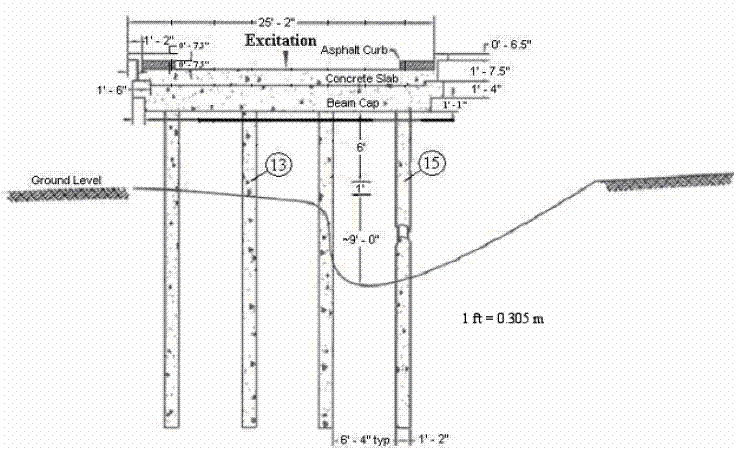Figure 109. Diagram. Vibration tests of bent 12 of the Trinity River Relief Bridge.

### Fourier and Wavelet Analysis Limitations for Dynamic Properties of Bent 12

Both Fourier and wavelet analysis techniques were applied to bent 12. Following is a description of the results.

### Global Modal Properties from Fourier Analysis

The most widely used method to identify global structural dynamic properties such as fundamental natural frequency and mode of a structure is Fourier spectral analysis of vibration data measured at limited locations. Figure 110 shows the time histories of vertical excitation (or forcing function) and its corresponding vertical (along-column) acceleration-vibration response at accelerometer 15 with bent 12 in its intact state. Both time histories are highly nonstationary, with the frequency increasing almost linearly from 5 Hz at 0.5 s to 75 Hz at 5.5 s. The Fourier spectra corresponding to the time-domain traces of figure 110 are shown in figure 111. The ratio of the Fourier spectrum of vibration in the middle graph of figure 111 to the Fourier spectrum of the forcing function in the top graph is the normalized Fourier spectrum, or the accelerance TF, of the bottom graph. The normalized Fourier spectrum shows the resonances clearly.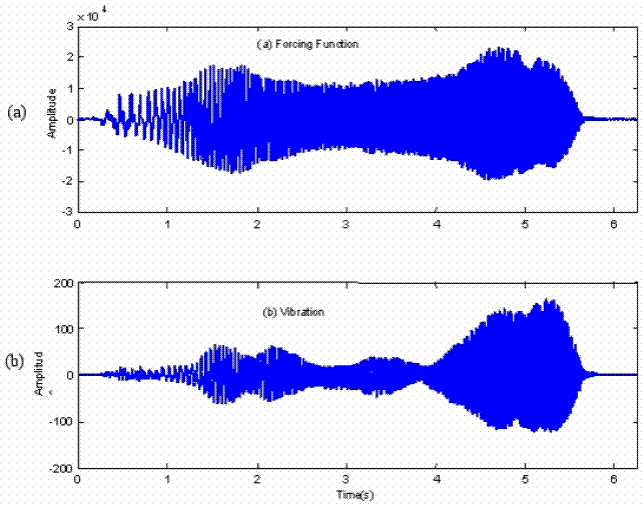Figure 110. Graphs. (a) Vibroseis chirp forcing function in poundforce at center of bent 2.(b) Accelerometer 15 response in inches/seconds squared.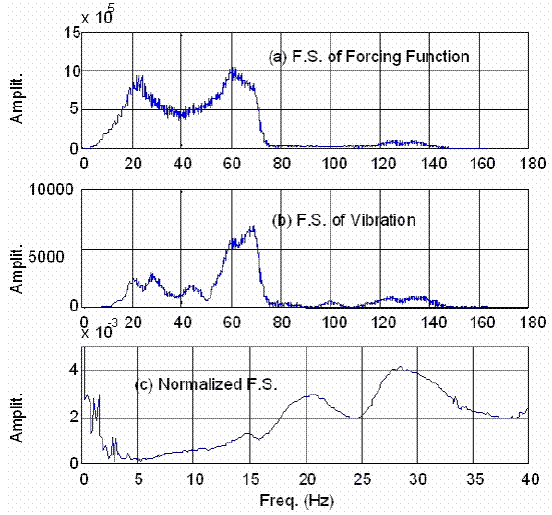Figure 111. Graphs. Fourier spectra (F.S.) of figure 110 data.

For example, the fundamental natural frequency of the structure is obtained by identifying the first peak location in the Fourier spectrum of vibration acceleration normalized by the Fourier spectrum of forcing function (this is the accelerance TF discussed in chapter 5), which is 14.8 Hz, as shown in figure 111. Note that the identified fundamental natural frequency (14.8 Hz) is close to the driving frequency content of the excitation time history during 1 to 2 s in figure 110a. Because the vibration time history between 1 and 2 s in figure 110b should contain a mixed content of both the driving and natural frequencies, among others, such a phenomenon exists only locally in 1 to 2 s, not globally in 0 to 6 s in the vibration time history. Therefore, the natural frequencies identified from the global-based (or time-independent) Fourier spectra may not distinguish the mixed frequency content well. The fundamental problem with this approach is the inability of the TF to clearly resolve, from the overall structural response, nonlinear responses at resonance associated with an individual damaged member.

Windowed Fourier spectral analysis could find window-length-dependent frequencies; however, windowed Fourier spectral analysis would not help with the dilemma of time-frequency resolution, that is, getting accurate low-frequency information at the cost of sacrificing the local time information and vice versa, as discussed earlier. Primarily because of this, no windowed Fourier spectral analyses were done.

### Global Modal Properties from Wavelet Analysis

Alternatively, wavelet analysis could be used to analyze the bent 12 data and thus recover timedependent information from the data, especially through the temporal-frequency energy distribution of vibration or simply the wavelet spectrum. Figure 112 center and bottom, respectively, present the wavelet spectra of the forcing function in figure 110 (replotted in figure 112 top) created with the use of mother wavelets described by Morlet(172) and Daubechies.(170) The latter characterizes his procedure as the creation of Db5 wavelets. These results clearly indicate that each of the wavelet spectra depends on the selected mother wavelet. For example, there exists only one dominant scale-decreasing trend of high-energy (white color in figure 112 center) as time increases from 0 s to 1,000 s in the Morlet(172) wavelet spectrum. This implies that the forcing function has a dominant frequency increasing from that time period because the frequency is inversely proportional to the scale. In the same period, there are three such trends in the Db5 wavelet spectra in figure 112 bottom. A similar phenomenon is also observed at the time period between 1,400 s to 1,800 s. More important, Morlet and Db5 mother wavelets have their own frequency band in the conventional frequency sense, that is, Fourierbased.(172) Therefore, the scale factor in the wavelet spectrum will no longer be simply (orinversely) related to the conventional Fourier-based frequency. Converting the scale in wavelet spectrum to the conventional Fourier-based frequency content requires much detailed analysis, and it is inefficient. This dependency on the wavelet selection is problematic for this study.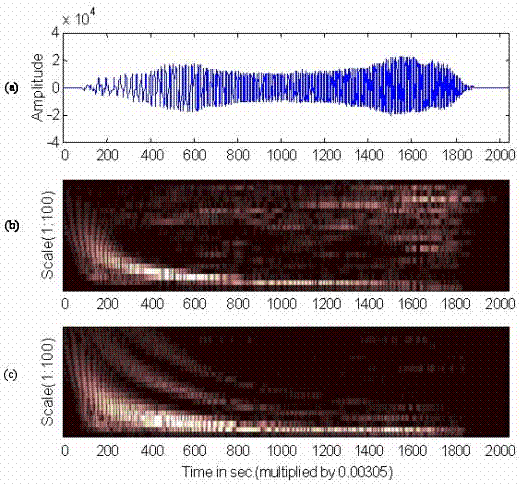Note: the lighter the color in graphs (b) and (c), the larger the energy in the wavelet spectra.

Figure 112. Graphs. Morlet (graph b) and Db5 (graph c) wavelet spectra of the forcing function (graph a).

### Local Properties

In response to an excitation or driving force, vibration measured at any point on a structure should reveal certain frequency information such as driving frequency content, natural frequency content of the whole structure and its members, and noise frequency content. If one structural member has been damaged&mdash;for example, the south pile only out of the four piles of bent 12 (see figure 109)&mdash;the stiffness and thus the natural frequencies, proportionally to the degree of the damage of the member, are reduced in comparison to those without damage. The vibration recorded on the damaged member could show larger vibration amplitude at lower frequency than the vibration at the same member without damage, as reported in chapter 5. That is the signature of damaged structures in vibration records collected near the damaged element. Damage may reduce the stiffness of a single member in a large structure; however, damage might not affect the stiffness of the whole structure significantly. Consequently, vibration measured on members other than the damaged one might not be sensitive to a small amount of localized damage in the damaged one; therefore, while the intact, excavated, and damaged pile states of bent 12 should produce three explicitly different signatures of local dynamic properties from vibration data taken at accelerometer location 15 on the damaged pile, corresponding data for the three states taken at accelerometer location 13 on an undamaged pile should produce three similar signatures.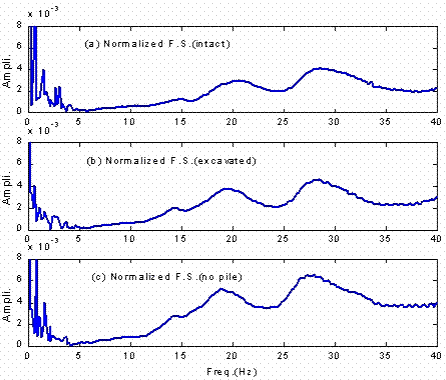Figure 113. Graphs. Accelerance TFs for intact, excavated, and broken pile states.

Figure 113 shows the accelerance TFs at accelerometer 15 for the intact, excavated, and broken pile states. The fundamental natural frequencies measured at accelerometer 15 in the excavatedand broken-pile states are only 6 percent and 9 percent lower than the natural frequency in the intact state. This probably can be explained by considering the difference between localized and global effects. The fundamental natural frequencies measured are of a global nature. Because the damage of bent 12 was localized, the global properties, even though measured at the damaged pile, were not changed much. Considering the difficulty of isolating local and global effects, it may be difficult in practice to tell if the change in a TF response is caused by structural damage, measurement error, or other reason such as temperature change, until a comprehensive database is developed.

### Hilbert-Huang Transform Analyses of Vibration Data from Bents 12 and 2

Recently, because of its uniqueness in faithfully representing nonlinear, nonstationary data, the HHT method has found many different and diverse applications, including use in blood-pressure variation, earthquake engineering, seismology, earthquake data analysis, building structural identification, building damage detection, and bridge system identification, among others. (See references 173, 174, 175, 176, 177, 178, 179, 180, 181, 182, 183, 184, and 185.) The following paragraphs discuss HHT fundamentals, followed by HHT analysis results for bents 12 and 2.

### Fundamentals of HHT Method

The HHT method consists of empirical mode decomposition (EMD) and Hilbert spectral analysis (HSA), which are summarized from Huang et al.(5,186)

### Empirical Mode Decomposition

Contrary to almost all existing methods of vibration data frequency analysis, EMD is based on the simple assumption that any data set consists of different, simple, intrinsic modes of oscillation that need not be sinusoidal. The nonsinusoidal character of each mode of oscillation is derived from the data. At any given time, the data may have many different coexisting modes of oscillation. The superposition of all these modes is the original data record. Each of these oscillatory modes, called an intrinsic mode function (IMF), is defined by the following conditions:

• Over the entire data set, the number of extrema and the number of zero-crossings must be equal or differ at most by one.
• At any point, the mean value of the envelope defined by the local maxima and the envelope defined by the local minima is zero.

An IMF represents a simple oscillatory mode similar to a component in the Fourier-based simple harmonic function, but it is more general. Any data can be decomposed as follows: First, identify all the local extrema; next, connect all the local maxima by a cubic spline to produce the upper envelope; and repeat the procedure for the local minima to produce the lower envelope. In this sifting process, the upper and lower envelopes should encompass all the data between them. Their mean can then be found as a function of time. The difference between the data and the mean is the first IMF component, which contains the finest scale or the shortest period component of the signal. This sifting process might be repeated a couple of times in practice. The first IMF component then can be removed from the data to obtain the residue, which is treated as the new data and subjected to the same sifting process as described above. This procedure can be repeated to obtain all the IMF components. The sifting process can be terminated if either the last IMF component or the residue is less than a predetermined value of consequence, or if the residue becomes a monotonic function from which no more IMF can be extracted. If the data have a trend, the final residue should be that trend. Mathematically, the data X can be given by the equation in figure 114.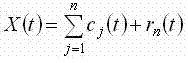Figure 114. Equation. X(t).

Where Cj is the jth component, rn is the residue, and (t) is time.

### Hilbert Spectral Analysis

Data C(t) and its Hilbert transform Y(t) can be combined to form the analytical signal Z(t), as in the equation in figure 115.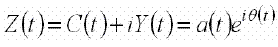Figure 115. Equation. Z(t).

Where a(t) = &radic;C2(t) + Y2(t) is the time-dependent amplitude and i&theta;(t) = arctan[Y(t)/C(t)] is the phase.

Applying the Hilbert transform to the n IMF components, the original data X(t) can be written in the form of the equation in figure 116.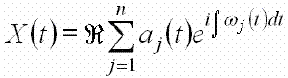Figure 116. Equation. X(t) in Hilbert transform form.

Where R denotes the real part of the value to be calculated and &omega;j(t) = d&theta;j(t)/dt is the instantaneous frequency of the jth IMF component.

The residue rn is not included in the equation in figure 116 because of its monotonic properties.The equation in figure 116 gives both amplitude and instantaneous frequency as functions of time. This equation is qualitatively different than the Fourier series representation in the equation in figure 117, which uses time-independent amplitude and phase.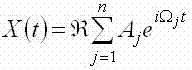Figure 117. Equation. X(t) in Fourier series representation.

Where R denotes the real part of the value to be calculated and Aj is the Fourier transform of X(t); &Omega;j is time-independent frequency.

A comparison of the equations in figures 116 and 117 suggests that the HHT represents a generalized Fourier expansion. Note that the windowed Fourier representation cannot give an instantaneous frequency because the smallest window length is governed by the data-sampling rate and the uncertainty principle. The time-dependent amplitude and instantaneous frequency in the equation in figure 116 not only improve the flexibility of the expansion but also enable the expansion to accommodate nonstationary data. The frequency-time distribution of the amplitude aj(t) is designated as the Hilbert amplitude spectrum H(w,t), or simply Hilbert spectrum. Its square reveals the evolutionary energy distribution or energy density.

Any complicated data set can be decomposed through EMD into a finite and often small number of IMFs that admits a well-behaved Hilbert transform. The EMD is based on the local characteristic time scale of the data; thus, it is adaptive to nonstationary data processes. The HSA defines an instantaneous or time-dependent frequency of the data through the Hilbert transform on each IMF component. The two steps of data processing discussed above, EMD and HSA, which combined are HHT analysis, show a generalized version of Fourier expansion. These two unique properties also enable the HHT analysis to reveal, as an alternative to Fourier-based or wavelet components and spectra, a possible physical meaning of each EMD-based IMF component and HSA-based Hilbert spectrum. The details of the method can be seen in Huang et al.(5,186)

### HHT Analysis of Data from Bent 12

The HHT analysis was first applied to the data from bent 12 because this bent had the simplest substructure geometry. Figures 118 and 119 depict contour plots for the HHT spectrum of the excitation and vibration time histories shown in figures 110a and 110b. The plots show the temporal-frequency energy distribution of the excitation and vibration signals. The darker colors in the figures indicate greater energy at that frequency and time. Figure 118 shows that the chirp excitation from the Vibroseis, which was applied at the center loading point, has a dominant energy band the frequency of which increases with time&mdash; at 0.5 s, the energy band is at 5 Hz; at 5.5 s, the energy band is at 75 Hz. The dominant frequency content is referred to in this paper as the dominant increasing driving frequency, or DIDF. The excitation also has energy at additional time-frequency combinations. Probably most notable, a grouping of frequencies ranging from approximately 20 Hz to 45 Hz is found between 0.5 and 1 s. This grouping can be inferred from the excitation time history shown in figure 110a.

According to vibration theory, the frequency content of the vibration response should contain primarily both the driving frequencies, such as shown in figure 118, and the natural frequencies of the structure. This assertion appears verified by figure 119, which shows the energy of the vibration response at accelerometer 15 for the intact bent. The main frequency content increases linearly with time, which corresponds to the behavior of the DIDF in figure 118. In addition to the energy that tracks the DIDF, figure 119 includes other energy concentrations, including a prominent grouping in the frequency range of 10 to 20 Hz between 1 and 2 s. These energy concentrations can be interpreted as the energy contributed from the structural vibration modes at various natural frequencies. Collectively, these natural frequencies constitute the signature of the structure. The prominent energy grouping in the frequency range of 10 to 20 Hz between 1 and 2 s was likely the result of the low frequencies of the driving force between 0.5 and 1 s. As time increased beyond 2 s, lower natural frequencies died down because of damping or energy levels too small in comparison with the strong DIDF energy to register in the analysis.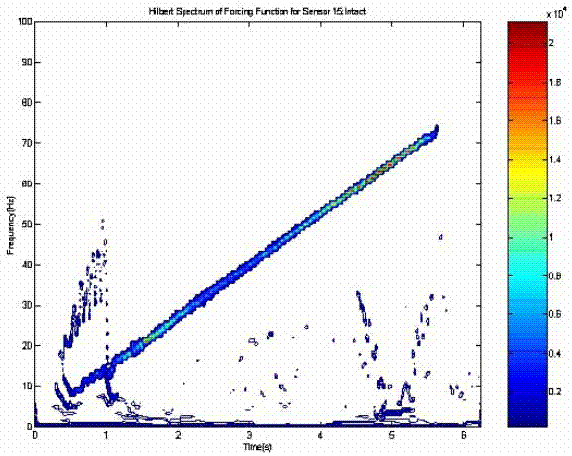Note: the darker the color, the larger the energy in the spectra.

Figure 118. Graph. HHT spectrum of forcing function, bent 12, intact state.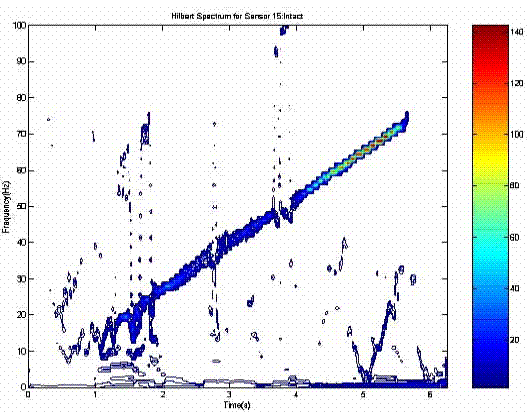Figure 119. Graph. HHT spectrum of vibration, accelerometer 15, bent 12, intact state.

To further demonstrate this point, examine the IMF components extracted from the vibration response and their corresponding Fourier spectra, shown respectively in figures 120 and 121. As seen from these figures, the first two or three IMF components are dominant because their amplitudes are much larger than other IMF components. The first IMF component contains the vibration with a frequency content linearly increasing with time, which could be explained as the vibration component primarily inherited from the Vibroseis excitation at the DIDF. The temporal-frequency energy distribution (the HHT spectrum) of the first IMF component in figure 122 further supports this clarification. The second and third IMF components contain relatively low frequency content, implying that they likely are the results of a couple of lowfrequency vibration modes. Their Fourier-based dominant frequencies (around 16 and 10 Hz) shown in figure 121 also partially support such a hypothesis. It appears that the fundamental natural frequency can be found from the HHT spectrum of the second or third IMF component, particularly around the time between 1 and 2 s. Figure 123 shows the HHT spectrum of the second IMF component, and it reveals that the lowest frequency of high energy in the time between 1 and 2 s is around 10 Hz.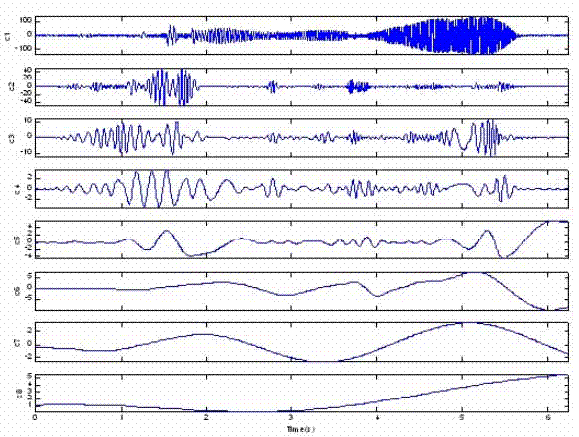Figure 120. Graphs. EMD with the eight IMF components of vibration, accelerometer 15, bent 12, intact state.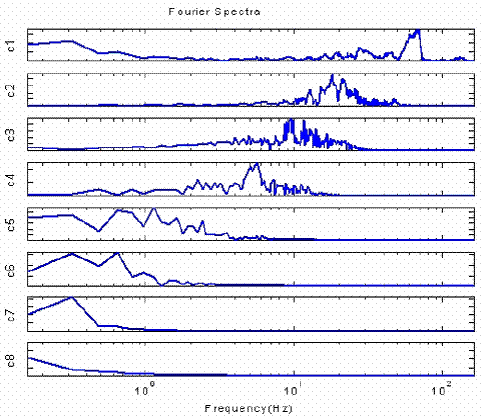Figure 121. Graphs. Fourier spectra of IMF components in figure 120.

HHT theory and software have the issue of mode mixture in IMF components. This is observed in the first and second IMF components in figures 123 and 124, in which part of the motion in the second IMF component should belong to the first component and vice versa. This issue can be partially, if not completely (because of its complex nature), solved by using the intermittency criterion or check in the HHT software. With the proper use of the intermittency check, all the modes can be separated with more IMF components generated. Such a sifting process with intermittency check will not significantly affect the whole temporal-frequency HHT spectrum. As a matter of fact, great progress recently has been made in refining the HHT in theoretical maturity and numerical accuracy in general and in unique decomposition of the data into IMF components in particular.(187) These refinements can overcome some drawbacks exposed in the early version of HHT software. Because identifying frequency content from HHT spectrum is of sole interest for the problem at hand, the intermittency check was not used at this stage of the study. The sifting process with intermittency check, however, is a possibility for examining in greater detail each IMF component.(182)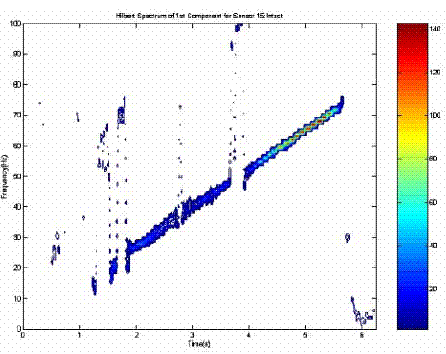Figure 122. Graph. HHT spectrum of first IMF component of vibration, accelerometer 15, bent 12, intact state.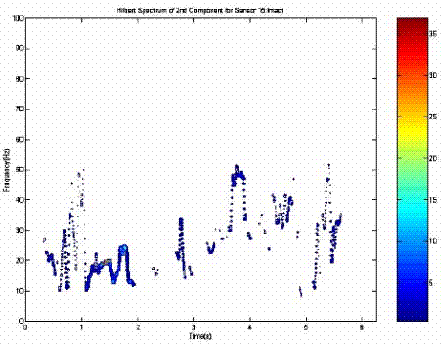Figure 123. Graph. HHT spectrum of second IMF component of vibration, accelerometer 15, bent 12, intact state.

Identifying the fundamental natural frequencies of the structure from the vibrations during the time between 1 and 2 s is of primary interest; consequently, most of the remaining figures containing the HHT spectrum are presented with times of less than 3 s and frequencies less than 40 Hz. Figure 124 enlarges the HHT spectrum of vibration response at accelerometer 15 with the structure in its sound state. Except for the DIDF, the energy concentration shown in figure 124 is attributed primarily to the structure itself. Because the accelerance TF shows a resonance of about 15 Hz, examination of the energy at frequencies up to 15 Hz during the first 1 to 2 s may be useful. The energy below roughly 10 Hz (light lines or dots) is much less than that above 10 Hz (dark lines or dots). This response energy is believed to be generated by the DIDF during the time between 0.5 and 1 s and the driving frequency of 6 to 15 Hz at around 1+ s, as shown in figure 118. If the fundamental natural frequency falls in the driving frequency range of 5 to 15 Hz, the response energy at the fundamental natural frequency should be much stronger than at lower frequencies, but not necessarily stronger than at higher frequencies because of the existence of second and higher natural frequencies. The dark area of 14 to 15 Hz at 1.5 s in figure 124 suggests that the fundamental natural frequency is approximately 14 to 15 Hz; but also, considerable energy is found from 12 to 20 Hz in the 1 to 2 s timeframe.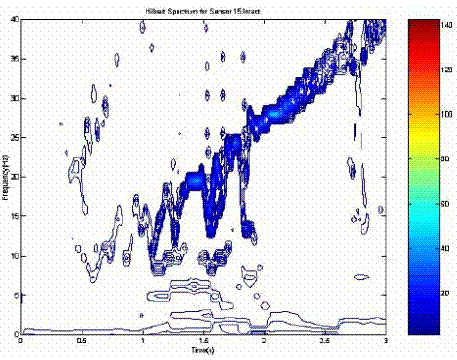Figure 124. Graph. HHT spectrum of vibration, accelerometer 15, bent 12, intact state.

Figure 125 shows the HHT spectrum of vibration response at accelerometer 15 with the structure in its excavated damage state. In addition to the DIDF, an energy concentration is found at approximately 7 Hz at approximately 1.4 s. This concentration corresponds to the concentration found at 14 to 15 Hz at 1.5 s in figure 122 for the intact pile. The decrease from 14 to 15 Hz in the intact state to 7 Hz in the excavated damage state could be related to the mixed natural frequency of the pile and pier because the vibration at accelerometer 15 should reflect the dynamic characteristics of both the whole structure and the local member. The excavated soil reduces the stiffness of the south pile and south-center pile, and thus the fundamental natural frequency of bent 12. This is the signature of local damage in the vibration records, damage that was not as clearly shown in traditional modal vibration data analysis. Such an explanation is further supported by figure 126, which shows the HHT spectrum of vibration response at accelerometer 15 with the south pile broken, and thus the structure in its broken state. The frequency concentration has further decreased to 3 Hz at 1.7 s.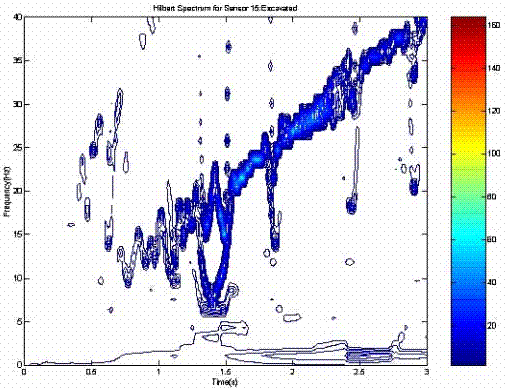Figure 125. Graph. HHT spectrum of vibration, accelerometer 15, bent 12, excavated state.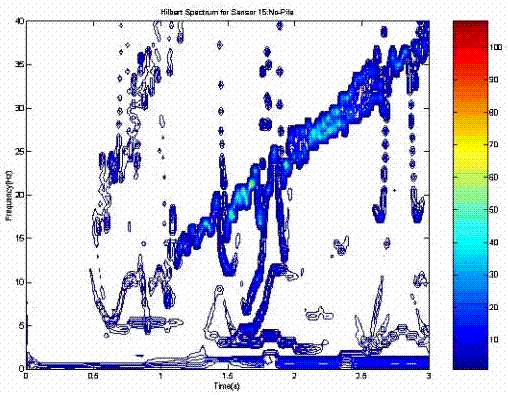Figure 126. Graph. HHT spectrum of vibration, accelerometer 15, bent 12, broken state.

The preceding discussion is based on the HHT-analyzed data observations with the aid of vibration theory. Further model-based validation is the subject of a continuing study. Following is a simple, rough argument about the validity of the preceding discussion.

Accelerometer 15 is mounted on the pile/column with one end connected to the beam and the other end buried in the soil; thus, the dominant vibration recorded should more likely contain the dynamic features of the column itself. To examine the change of fundamental natural frequency of the column in three damage states, one can roughly model the column with different boundary conditions and lengths. In particular, the boundary conditions are approximated as fixed-fixed with a length of 2.14 m (7 ft) for the pile/column in the intact state, fixed-fixed with a length of 4.88 m (16 ft) in the excavated damage state, and fixed-free with a length of 2.14 m (7 ft) in the broken pile/severe damage state. These boundary conditions were chosen not only because they crudely model the real vibration of the column but also because they have simple exact solutions for the fundamental natural frequency. This simple theoretical model shows that the relative reduction of the fundamental natural frequency for the excavated and broken pile states with respect to that in the intact state is 56 and 68 percent, respectively. In comparison, the pertinent relative change with the use of the observed fundamental natural frequency is 50 and 70 percent. The detailed comparison is given in table 15. The observed results are close to the theoretical ones. While this comparison is not proof that this discussion is a correct approach to analyzing the data, it does provide substantial support for the approach. Further research and solid validation are needed.

Table 15. Comparison of fundamental natural frequencies of a column in three states.

StateBoundary Condition with LengthTheoretical Frequency (F)Observed Frequencies (HHT Analysis) (f)Relative Change of Frequency with Respect to Frequency at Intact StageFigure
IntactFixed-fixed with l = 2.1 m (7 ft)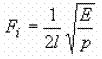fi = 10 HzN/A127
Minor damageFixed-fixed with l = 4.9 m (16 ft)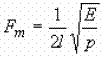fm = 5 Hz(Fi-Fm)/Fi = 56%(fi-fm)/fi = 50%128
Severe damageFixed-free with l = 3.4 m (11 ft)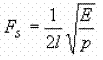fs = 3 Hz(Fi-Fs)/Fi = 68%(fi-fs)/fi = 70%129

The HHT analysis of the three states of vibration at accelerometer 15 on bent 12 shows that the HHT method might provide a means to recognize clearly, in terms of the natural frequency as determined from vibration records, the signature difference of sequential damage in a structural member. As a comparison, an HHT analysis of corresponding vibration at accelerometer 13 on the north-center pile, which is well-removed from the damage location at the south pile, is now presented.

Figures 127, 128, and 129 show the HHT spectrum of vibration data at accelerometer 13 for the structure in its intact, excavated, and broken or damaged pile states, respectively. The figures show that the lowest dominant frequency of structural vibration is approximately 10 Hz between 1 and 2 s. These data not only confirm that the fundamental natural frequency of the whole structure and its members likely can be distinguished from the driving frequency by HHT analysis, but they also suggest that HHT analysis is sensitive to changes in vibration characteristics caused by local damage.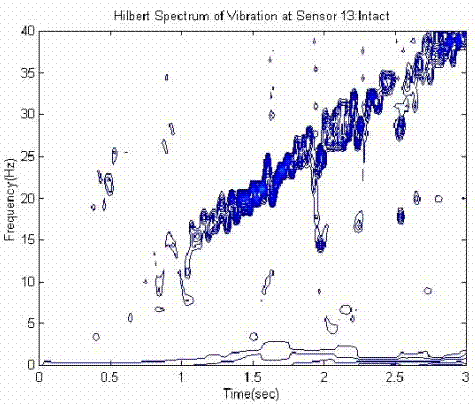Figure 127. Graph. HHT spectrum of vibration, accelerometer 13, bent 12, intact state.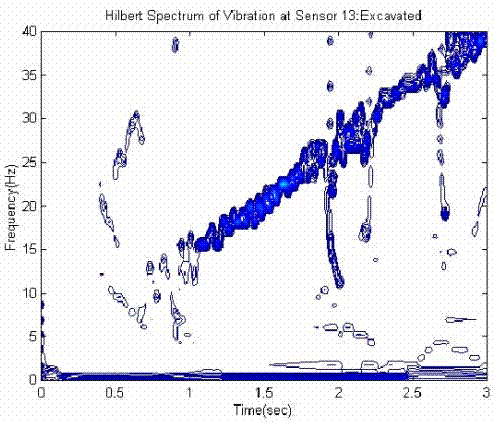Figure 128. Graph. HHT spectrum of vibration, accelerometer 13, bent 12, excavated state.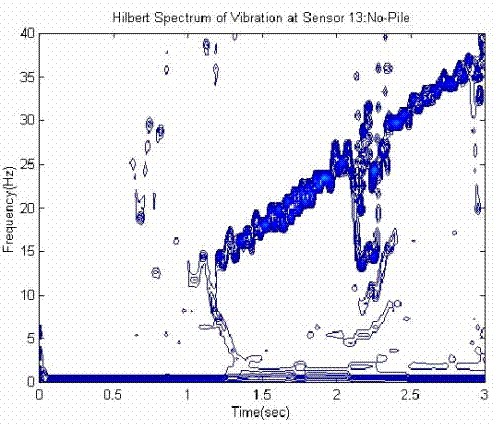Figure 129. Graph. HHT spectrum of vibration, accelerometer 13, bent 12, broken state.

The second and third IMF components of vibration, or at least the second, are most related to the structural fundamental frequency of vibration. The HHT spectra of second IMF components of vibrations at accelerometers 15 and 13 with the structure in its intact, excavated, and damaged pile states are shown in figures 130 through 135. The mode mixture of IMF components is observed (which results in the mixture of driving and natural frequencies). The figures also show the response differences between accelerometer 15 on the excavated and then broken south pile and accelerometer 13 on the sound north pile as the south pile goes from the intact, to the excavated, to the broken state. At accelerometer 15, the fundamental frequency appears to decrease from 10 Hz, to 7 Hz, to 5 Hz. In contrast, the fundamental frequency at accelerometer 13 shows little movement.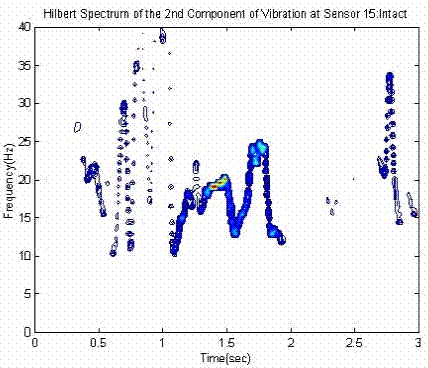Figure 130. Graph. HHT spectrum, second component of vibration, accelerometer 15, bent 12, intact state.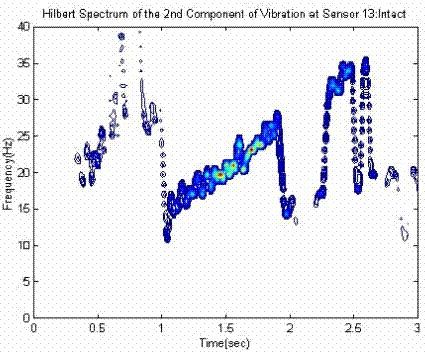Figure 131. Graph. HHT spectrum, second component of vibration, accelerometer 13, bent 12, intact state.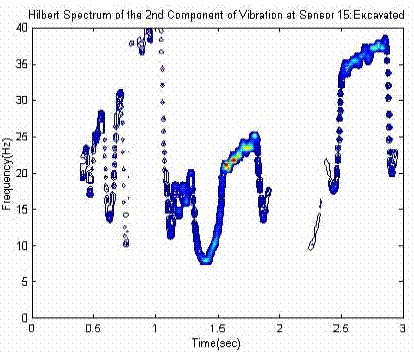Figure 132. Graph. HHT spectrum, second component of vibration, accelerometer 15, bent 12, excavated state.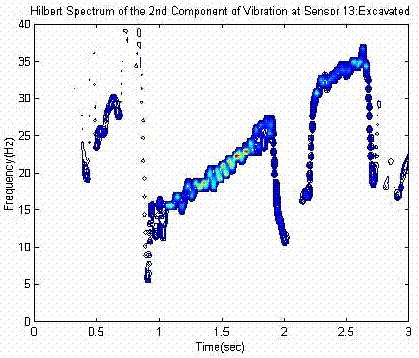Figure 133. Graph. HHT spectrum, second component of vibration, accelerometer 13, bent 12, excavated state.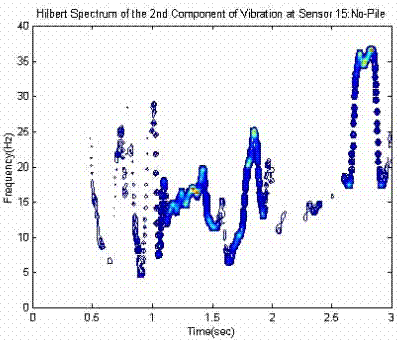Figure 134. Graph. HHT spectrum, second component of vibration, accelerometer 15, bent 12, broken state.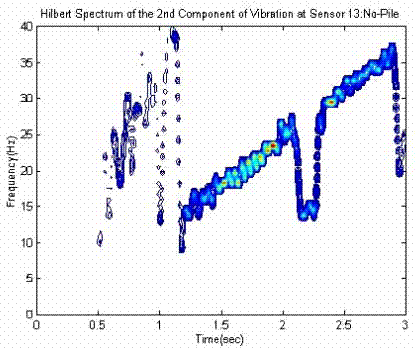Figure 135. Graph. HHT spectrum, second component of vibration, accelerometer 13, bent 12, broken state.

### HHT Analysis of Data from Bent 2

The following paragraphs discuss the HHT analysis of the data from bent 2. The focus is on the potential of HHT analysis for recognizing damage. Figure 136 shows the structural configuration of bent 2 in its broken pile state. The Vibroseis excitation was in the middle of the deck. Bent 2 differs from bent 12 because bent 2 had a ground-level shallow footing surrounding the four piles of the bent. Other pier and test conditions, including the field test setup, data collection procedures, and states of damage, were similar to the conditions in the testing of bent 12. The modal vibration test data were analyzed from accelerometers 11 and 9 on two of the four bent 2 piles, shown in figure 136. The accelerometers were located somewhat higher on the piles than accelerometers 15 and 13 on the piles of bent 12.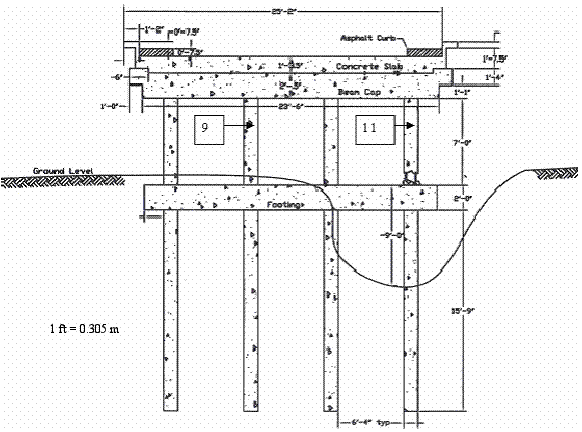Figure 136. Diagram. Locations of accelerometers 9 and 11 on bent 2.

Figures 137, 138, and 139 show the HHT spectra of the vibration response at accelerometer 11 on the damaged pile with the structure in its intact, excavated, and damaged pile states. Figures 140, 141, and 142 show the HHT spectra of the vibration response at accelerometer 9, which is on a sound pile. Both sets of results are similar to the spectra of bent 12.

Between 1 and 1.5 s at the damaged pile, the vibration frequencies measured by accelerometer 11 drop from 10 Hz in the intact state to less than 5 Hz in the damaged state. Over the same time range at the undamaged pile, the vibration frequencies measured by accelerometer 9 show a slight increase in frequencies. These results tract those of the bent 12 case and support the conclusion that an HHT spectra from a damaged location is more sensitive to the damage than a spectra from a more remote location.

Thus the HHT method appears to provide a means to diagnose a damaged structural member through the examination of vibration signatures contained in a limited number of vibration records. It also appears that sensors need to be fairly close to or on a damaged member to monitor changes.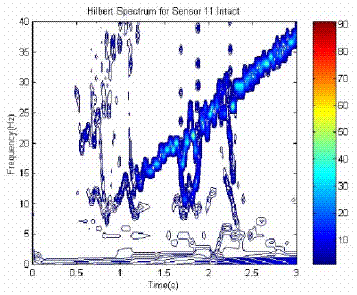Figure 137. Graph. HHT spectrum of vibration, accelerometer 11, bent 2, intact state.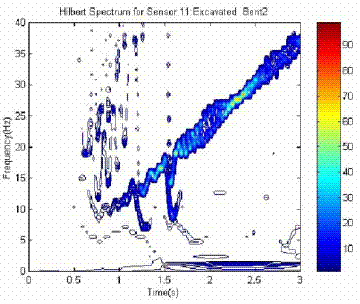Figure 138. Graph. HHT spectrum of vibration, accelerometer 11, bent 2, excavated state.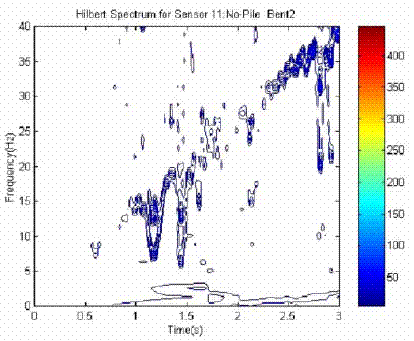Figure 139. Graph. HHT spectrum of vibration, accelerometer 11, bent 2, broken state.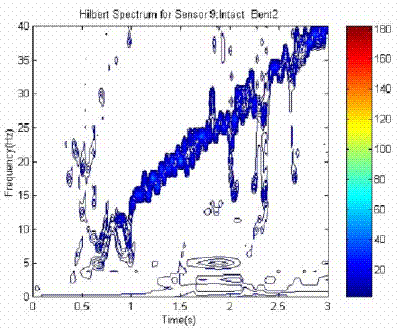Figure 140. Graph. HHT spectrum of vibration, accelerometer 9, bent 2, intact state.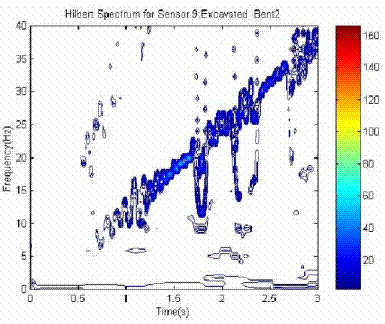Figure 141. Graph. HHT spectrum of vibration, accelerometer 9, bent 2, excavated state.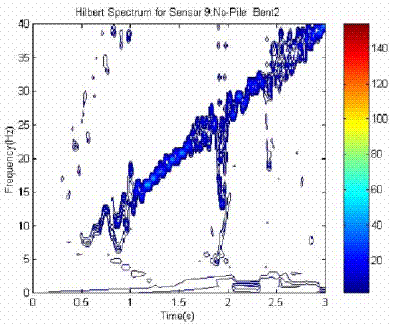Figure 142. Graph. HHT spectrum of vibration, accelerometer 9, bent 2, broken state.

### Force Excitation Normalized HHT Spectra for Bent 12

HHT analysis appears to be very useful in identifying the frequency content of linear and nonlinear signals. To investigate its usefulness further, a normalization approach was applied to the HHT analysis of bent 12 data, more specifically to readings at accelerometer 15 on the damaged pile on bent 12. This analysis was presented earlier. The normalization approach was similar to the modal accelerance TF analysis in that the HHT spectrum of the accelerometer response readings at accelerometer 15 were divided by the HHT spectrum of the Vibroseis chirp excitation force. In other words, the HHT accelerometer response readings were normalized to the HHT input force. The input force was the average amplitude at each digital time step over the 6-s measurement range. The input force, or chirp excitation force, was not constant with time, so normalization was an attempt to remove the effect in the response data of the time varying aspect of the input force. The hope was that normalization would make resonant frequency peaks and shifts more apparent. A further hope was that the readily apparent vertical resonance frequencies obtained from the modal vibration measurements and TF analyses discussed in chapter 5 and shown in figure 113 would have corresponding resonances in the normalized HHT spectrum.

Figure 118 was the source of the HHT-processed data for the averaging of the amplitudes of the input force. For the intact state case, figure 119 was the source of the HHT-processed response readings at accelerometer 15. Other figures provided the HHT-processed response readings for the excavated and broken pile cases.

The resulting HHT spectrum plots of the normalized vibration at accelerometer 15 for the intact, excavated, and broken pile cases are given in figures 143, 144, and 145, respectively. The average amplitudes of the input force&mdash;the dominant, linear chirp force&mdash;that was used to normalize the corresponding HHT spectra for the intact, excavated, and broken pile states are shown versus time in figures 146, 147, and 148, respectively. Over the 6-s time window, these HHT amplitude plots show considerable variation. The responses at accelerometer 15 should vary in amplitude with the excitation or input force except when the structural resonant frequencies are excited by the chirp, and dynamic amplification of the response occurs, indicating a resonant peak frequency. Figure 143 shows high amplitudes at 20 Hz and 28 Hz that correspond closely to the modal vibration peaks in figure 113 for the intact case of bent 12. The weaker modal resonance peak at 15 Hz, however, does not stand out in the normalized HHT spectrum in figure 143. The integration of the acceleration data to velocity and displacement data in the time domain before HHT analyses might have made the 15 Hz resonance more apparent. Agreement between the modal and normalized HHT resonances&mdash;the latter are in figures 145 and 147&mdash;is not apparent for the excavated and broken pile cases, possibly because of the damage and nonlinear effects.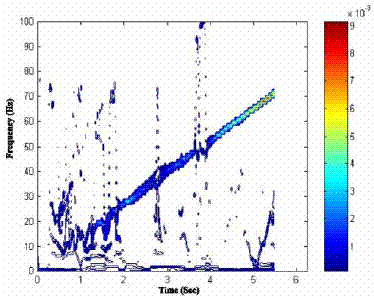Figure 143. Graph. Normalized HHT spectrum of vibration, accelerometer 15, bent 12, intact state.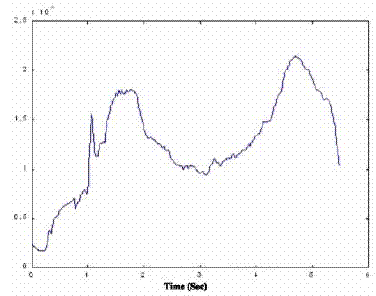Figure 144. Graph. Amplitude of force applied to bent 12, intact state.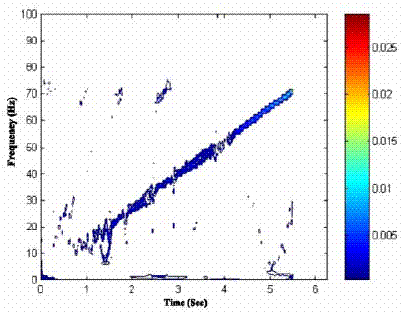Figure 145. Graph. Normalized HHT spectrum of vibration, accelerometer 15, bent 12, excavated state.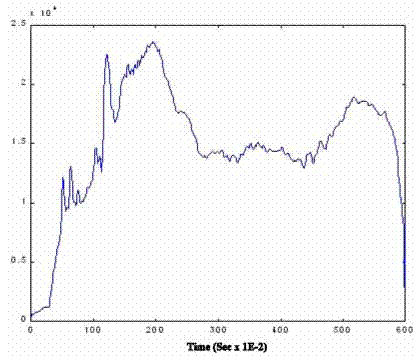Figure 146. Graph. Amplitude of force applied to bent 12, excavated state.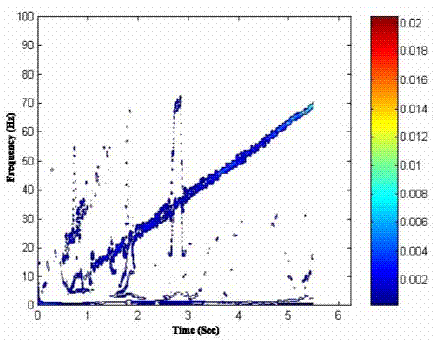Figure 147. Graph. Normalized HHT spectrum of vibration, accelerometer 15, bent 12, broken state.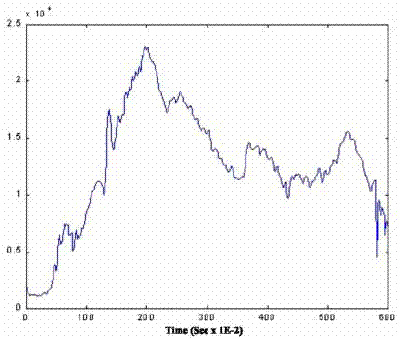Figure 148. Graph. Amplitude of force applied to bent 12, broken state.

### Model-Based Validation for Bent 12

To further investigate both the modeling approach covered in chapter 6 and the use of HHT analysis in determining damage characteristics, an HHT analysis was performed with simulated vibration data and ANSYS-based 2-D FEMs for bent 12 in its intact and severe-damage states. It should be noted that there are always inherent differences between the FEMs and the real structure. These differences arise from simplifications of boundary and support conditions, the connectivities between various structural elements, unknown material properties and constitutive relationships (particularly those associated with soil and concrete), and energy dissipation (damping) mechanisms. Only certain aspects of the HHT-based signature-recognition features were investigated with the model-based approach.

### ANSYS Model for Bent 12

The ANSYS-based 2-D FEM is shown in figure 150 for bent 12 in its intact state. The model was built on design specifications and pertinent information by Sanayei and Santini.(135) This 2-D model is also consistent with a 3-D FEM in terms of dynamic characteristics of the bent in the vertical direction.(135) Vibration in the vertical direction is the sole interest in this study, so the 2- D model instead of 3-D model was used for simplicity. In the 2-D modeling for bent 12, the cap beams and columns of the bent were modeled with beam elements and rigidly connected to each other. The mass and stiffness of the bridge deck were lumped onto the cap beam. The four piles were simply supported on the ground. Nodes 53 and 37 correspond to the locations of nodes 15 and 13 in figure 109. In the study, the 2-D model was extended from the intact state to the severe-damage state by releasing boundary restrictions at the ground end of the south pile (pile 15) in figure 150.

Table 16 shows the first 10 undamped natural frequencies of the intact and severe-damage states of the 2-D model of bent 12. The first, second, and third natural frequencies in the vertical direction are also indicated. To simulate the damping effects, Rayleigh damping was used in the modeling. The resulting equation is given in figure 149. The damping matrix [C] is expressed in terms of the matrices of mass [M] and stiffness [K].(188)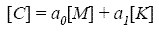Figure 149. Equation. Damping matrix [C].

Where a0 and a1 are the constant coefficients; for simplicity, a0 = a1 = d was used in the simulations.

The first three natural frequencies in the vertical direction in table 16 are neither consistent with those of the Fourier-based analysis nor with those of the HHT analysis. Moreover, the damping in the model might differ significantly from the damping experienced by the actual bridge. Nevertheless, the 2-D model is used to verify whether the observations and assertions for the HHT method is a way to identify the downward shift in instantaneous frequency associated with the excavated and broken pile states.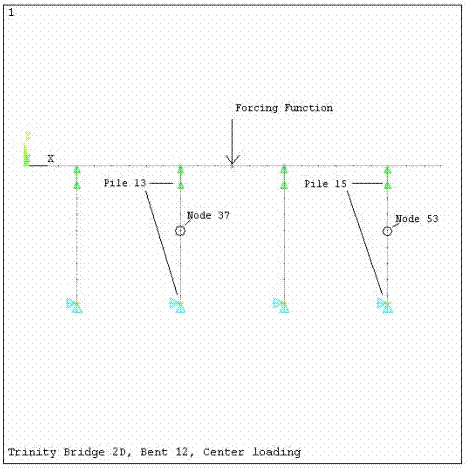Figure 150. Diagram. ANSYS model for bent 12.

Table 16. Fundamental natural frequencies of bent 12.

Natural Frequencies (Comments)Intact (Hz)Severe Damage (Hz) (Percent Decrease with Respect to Intact)
1st3.993.29
2nd (1st vertical natural frequency)11.986.95 (41.98%)
3rd14.8412.47
4th (2nd vertical natural frequency)19.6818.08 (8.13%)
5th44.0139.19
6th (3rd vertical natural frequency)69.2252.50 (24.15%)
7th93.8872.50
8th104.4595.00
9th106.37105.82
10th108.49108.17

### HHT Analysis of Simulated Data

The forcing function graphed in figure 110a was used to excite the 2-D model of figure 153 to calculate the vibration responses at nodes 53 and 37. Figures 151, 152, and 153 show the Hilbert amplitude spectrum of the theoretical model response at node 37 of the bent in the intact bent state with no damping, d = 0. The response energy is focused in frequency bands centered primarily around 12.0 Hz, 19.7 Hz, and 69.2 Hz. These are, respectively, the first three theoretical natural frequencies in the vertical direction, as shown in table 16. The marginal amplitude spectrum in figures 154, 155, and 156 clearly show the three resonant frequencies for the undamped case of d = 0.

Without damping, the bent is fully excited at the theoretical natural frequencies; therefore, the vibration energy at natural frequencies typically is higher than the driving excitation-inherited energy (with the natural frequency effects of the vibrator) at the chirp frequency. Consequently, with the exception of the strong energy band from 40 to 70 Hz between 3.5 and 5 s, the energy inherited from the excitation at chirp frequency is not clearly shown in figures 151, 152, and 153. With increasing damping, the relative intensities of the energy from the structure and excitation should be affected, which arguably is discernable in the Hilbert amplitude spectra in figures 152 and 153 for d = 0.00198 and 0.05305, respectively.

The theoretical marginal amplitude spectrum in figure 155 suggests that the first damped fundamental natural frequency in the vertical direction might be around 11.5 Hz, which is correctly at a lower resonance than for the undamped theoretical case at 11.98 Hz. (See table 16.) This result is consistent with vibration theory. Because of the large damping used in the theoretical calculations and the strong excitation at much of the chirp frequency range, the vibration energy at natural frequencies appears greatly suppressed, as seen in figures 151 and 152. The first mode vibration energy at 11.5 Hz in figure 155 might be comparable to the chirp energy at other frequencies.

Figures 153 and 156 show the largest theoretical damping. The vibration energy at 10 Hz appears to occur primarily at 1.4 s. Because the d = 0.05305 damping here is extremely high, the theoretically calculated damped fundamental natural frequency is greatly downshifted from the undamped value of 11.98 to 10 Hz. It should be noted that with damping of d = 0.05305, the ANSYS model in figure 150 generated time history responses similar to those of figure 110b. This implies that real damping of bent 12 in the vertical direction is comparatively high.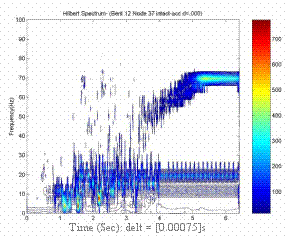Figure 151. Graph. HHT spectra of vibration of 2-D FEM, node 37, bent 12, intact state, d = 0.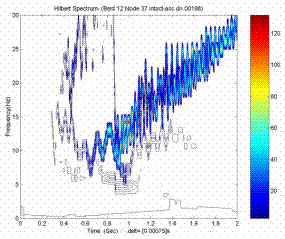Figure 152. Graph. HHT spectra of vibration of 2-D FEM, node 37, bent 12, intact state, d = 0.00198.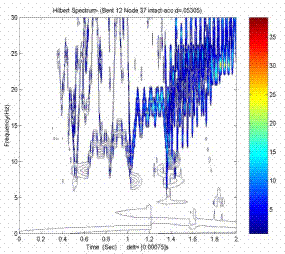Figure 153. Graph. HHT spectra of vibration of 2-D FEM, node 37, bent 12, intact state, d = 0.05305.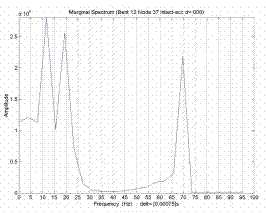Figure 154. Graph. Spectra of marginal amplitude, node 37, bent 12, intact state, d = 0.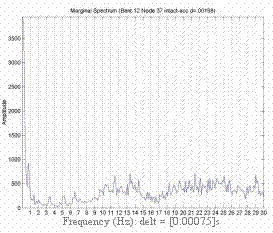Figure 155. Graph. Spectra of marginal amplitude, node 37, bent 12, intact state, d = 0.00198.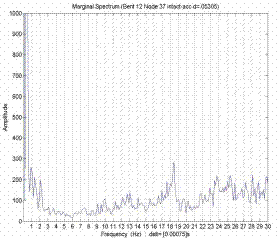Figure 156. Graph. Spectra of marginal amplitude, node 37, bent 12, intact state, d = 0.05305.

To examine theoretical results for the broken pile state, the Hilbert amplitude spectra of the response at node 37 on the sound pile and at node 53 on the broken pile are presented in figures 157 and 158 for the broken pile state and for d = 0.003979. In other words, the pile of node 53 in the model of bent 12 was theoretically broken, and readings were taken at node 53 on the broken pile and node 37 on the sound pile. The node 53 readings in figure 158 show the vibration energy concentrated at 7 Hz from 0.5 to 1.2 s, which is the fundamental natural frequency of the theoretical broken pile case in table 16. In contrast, the dominant resonant vibration energy at node 37&mdash;figure 157&mdash;is approximately 12 Hz between 0.6 and 1 s; a minor resonant peak is found at 7 Hz at just over 1 s.

The marginal amplitude spectra for the sound and broken piles are presented in figures 159 and 160, respectively.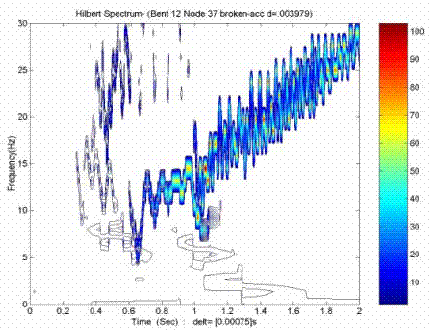Figure 157. Graph. HHT spectra of vibration of 2-D FEM, d = 0.003979, bent 12, broken state, node 37.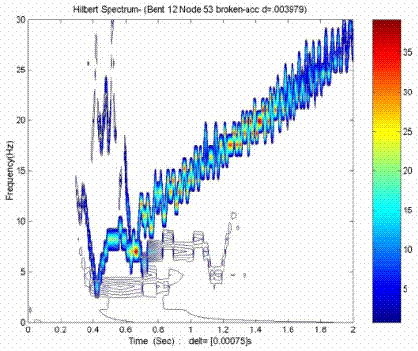Figure 158. Graph. HHT spectra of vibration of 2-D FEM, d = 0.003979, bent 12, broken state, node 53.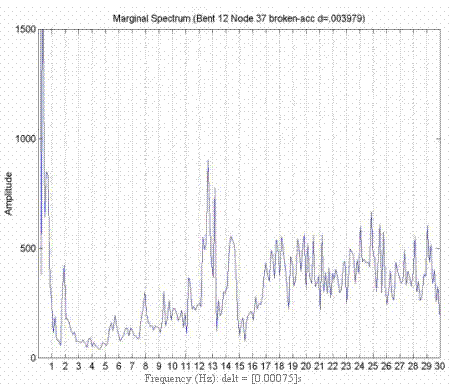Figure 159. Graph. Spectra of marginal amplitude, d = 0.003979, bent 12, broken state, node 37.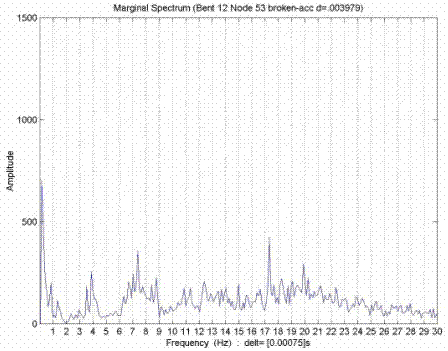Figure 160. Graph. Spectra of marginal amplitude, d = 0.003979, bent 12, broken state, node 53.

### Summary of Hilbert-Huang Transform Results

The preliminary, initial study discussed in this chapter compares the HHT view of vibration records of bridge structures in their intact, excavated, and damaged (broken) pile states with traditional modal vibration analyses. The comparison shows that HHT-based signature recognition might be a means of identifying local dynamic properties. In addition, HHT analysis might be a means of determining differences in the signatures of local structural members. The HHT approach appears to identify local, nonlinear, and lower frequency responses of structural members to damage. A new concept of normalizing HHT response spectrum data to HHT force excitation data was shown to have possibilities for revealing modal vibration resonances and removing the effects of varying force excitation. Finally, a comparatively simple FEM showed theoretically that the HHT method identified natural frequencies of undamped systems readily, and the damped theoretical result was similar to actual experimental data. Further validation of HHT analysis is necessary, but if the approach proves successful, the need for a priori data for damage diagnosis could be reduced or even eliminated.